# 计及日照与风速影响的户外气体绝缘母线温升计算Calculation of Temperature Rise of Outdoor Gas Insulated Buses Considering the Influence of Sunshine and Wind Speed

DOI: 10.12677/JEE.2019.73020, PDF, HTML, XML, 下载: 307  浏览: 1,024

Abstract: The running state of outdoor gas insulated buses is greatly affected by environmental factors, so the calculation model used for indoor equipment cannot be well applied. In this paper, a three- dimensional eddy current field analysis model is established for outdoor gas insulated buses to calculate the power loss of each part of the bus, and it is taken as a finite element analysis model for thermal load input fluid field and temperature field, and solar radiation model and wind speed model are added to calculate the temperature field distribution of the bus. The results show that sunshine and wind speed affect the temperature field distribution of the shell, which should be taken into account when analyzing the temperature field of the bus. The solar radiation model and wind speed model used in this paper have practical significance to calculate the temperature rise of outdoor equipment.

1. 引言

2. 户外气体绝缘母线物理模型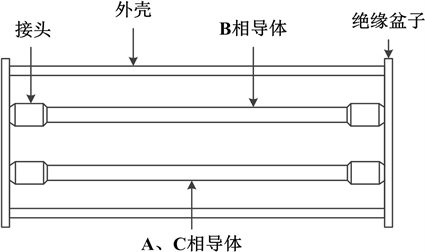Figure 1. Schematic diagram of gas insulated bus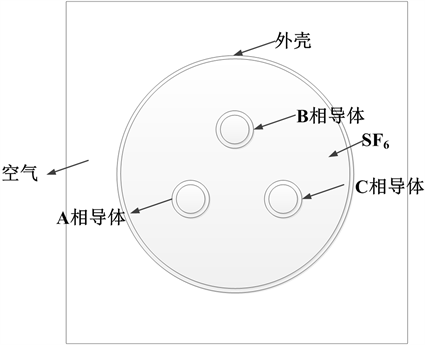Figure 2. Section diagram of gas insulated busTable 1. Basic geometric parameters of gas insulated bus

1) 母线内部电磁场属于似稳场，进行计算时不考虑位移电流的影响；

2) 母线三相导体内部电流为工频正弦电流，相位依次滞后120˚，频率为50 Hz；

3) 模型中三相导体、接头、外壳的相对磁导率为常数；

4) 导体、接头以及外壳电导率均随温度的变化而变化。

3. 三维涡流-流体-温度场建模

3.1. 涡流场控制方程与边界条件

$\nabla ×H=J$ (1)

$\nabla ×E=\frac{\partial B}{\partial t}$ (2)

$\nabla \cdot B=0$ (3)

$\nabla \cdot D=\rho$ (4)

$B=\mu H$ (5)

$D=\epsilon E$ (6)

$J=\sigma E$ (7)

$\nabla \cdot A=0$ (8)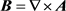(9)

${\nabla }^{2}A=-\mu J$ (10)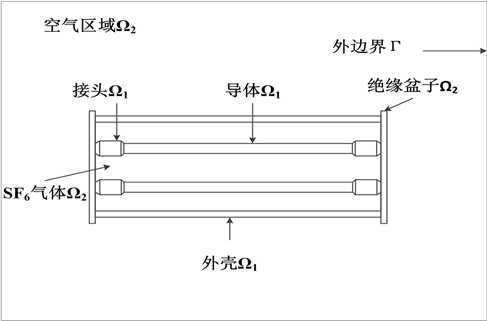Figure 3. Schematic diagram of solution domain of three-dimensional eddy current field for gas insulated bus

${A|}_{\Gamma }=0$ (11)

3.2. 三维流体-温度场耦合分析

3.2.1. 流体场控制方程

$\frac{\partial \rho }{\partial t}+\frac{\partial \left(\rho {V}_{x}\right)}{\partial x}+\frac{\partial \left(\rho {V}_{y}\right)}{\partial y}+\frac{\partial \left(\rho {V}_{z}\right)}{\partial z}=0$ (12)(13)

$\frac{\partial \left(\rho {V}_{y}\right)}{\partial t}+\nabla \cdot \left(\rho V{V}_{y}\right)=\nabla \cdot \left(\mu \nabla {V}_{y}\right)-\frac{\partial p}{\partial y}+\rho {f}_{y}$ (14)

$\frac{\partial \left(\rho {V}_{z}\right)}{\partial t}+\nabla \cdot \left(\rho V{V}_{z}\right)=\nabla \cdot \left(\mu \nabla {V}_{z}\right)-\frac{\partial p}{\partial z}+\rho {f}_{z}$ (15)

$\begin{array}{l}\frac{\partial }{\partial t}\left(\rho {C}_{p}T\right)+\frac{\partial }{\partial x}\left(\rho {v}_{x}{C}_{p}T\right)+\frac{\partial }{\partial y}\left(\rho {v}_{y}{C}_{p}T\right)+\frac{\partial }{\partial z}\left(\rho {v}_{z}{C}_{p}T\right)\\ =\frac{\partial }{\partial x}\left(\lambda \frac{\partial T}{\partial x}\right)+\frac{\partial }{\partial y}\left(\lambda \frac{\partial T}{\partial y}\right)+\frac{\partial }{\partial z}\left(\lambda \frac{\partial T}{\partial z}\right)+{Q}_{V}\end{array}$ (16)

3.2.2. 温度场控制方程

$q+\frac{\partial }{\partial x}\left({\lambda }_{x}\frac{\partial T}{\partial x}\right)+\frac{\partial }{\partial y}\left({\lambda }_{y}\frac{\partial T}{\partial y}\right)+\frac{\partial }{\partial z}\left({\lambda }_{z}\frac{\partial T}{\partial z}\right)=\rho c\frac{\text{d}T}{\text{d}t}$ (17)

$q=h\left({T}_{w}-{T}_{f}\right)$ (18)

$q=\sigma \epsilon {F}_{ij}\left({T}_{i}^{4}-{T}_{j}^{4}\right)$ (19)

1) 母线导体内部焦耳热损耗以热传导方式传递至导体表面；

2) 热量由导体表面通过自然对流的方式传递至SF6气体；

3) 热量分别由SF6气体与导体表面以自然对流与有限空间面-面辐射的方式进入母线外壳内表面；

4) 母线整体发热量由外壳内表面通过热传导传递至外壳外表面，与此同时太阳辐射热量通过日照的方式传递至外壳外表面；

5) 最后，外壳外表面全部热量以由于风速引起的强制对流与大空间辐射的方式耗散至周围空气中。

4. 太阳辐射模型与风速模型

4.1. 太阳辐射模型

${I}_{Di}={I}_{DN}\mathrm{cos}i={I}_{0}{P}^{m}\mathrm{cos}i$ (20)

${I}_{D\theta }={I}_{DH}\frac{1+\mathrm{cos}\theta }{2}=0.5{I}_{0}\mathrm{sinh}\frac{1-{P}^{m}}{1-1.4\mathrm{ln}P}{\mathrm{cos}}^{2}\frac{\theta }{2}$ (21)

${I}_{R\theta }={R}_{e}\left({I}_{DN}\mathrm{sinh}+{I}_{D\theta }\right){\mathrm{sin}}^{2}\frac{\theta }{2}$ (22)

$I={I}_{Di}+{I}_{D\theta }+{I}_{R\theta }$ (23)

$I={\alpha }_{s}{I}_{s}$ (24)

4.2. 风速模型

0~12级风力对应的风速如表2所示 。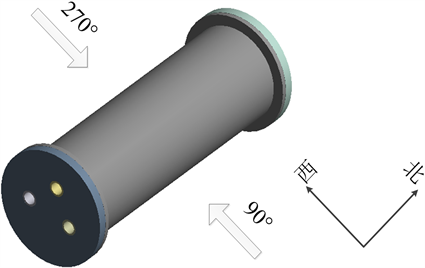Figure 4. Wind Direction Diagram

4.3. 边界条件

1) 在母线内外流固耦合交界面指定无滑移边界条件，即流体的相对速度为0，即：

${v}_{x}={v}_{y}={v}_{z}=0$ (25)

2) 在求解域外边界指定环境温度值，即第一类边界条件，该温度ft(t)可随时间t以及位置变化以模拟实际运行母线中环境温度变化为：

$T={f}_{t}\left(t\right)$ (26)

3) 在SF6气体与三相导体、外壳交界面施加面-面辐射换热的边界条件  为：

$-\lambda \frac{\partial T}{\partial n}={\epsilon }_{e}{\sigma }_{s}{F}_{ij}\left({T}_{c}^{4}-{T}_{t}^{4}\right)$ (27)

4) 户外气体绝缘母线外壳表面受到太阳直射，因此外壳表面传热边界条件可以表述为：

$-\lambda \frac{\partial T}{\partial n}={q}_{s}$ (28)

5) 户外气体绝缘母线考虑风速影响，在求解域外边界施加风速入口边界条件，即：

$v={v}_{0}$ (29)

4.4. 计算流程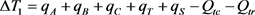(30)

$\Delta {T}_{2}={q}_{A}+{q}_{B}+{q}_{C}-{Q}_{cc}-{Q}_{cr}$ (31)

$\left\{\begin{array}{l}\Delta {T}_{1}/{T}_{01}<3%\\ \Delta {T}_{2}/{T}_{02}<3%\end{array}$ (32)

5. 计算结果与分析

5.1. 日照对温度场的影响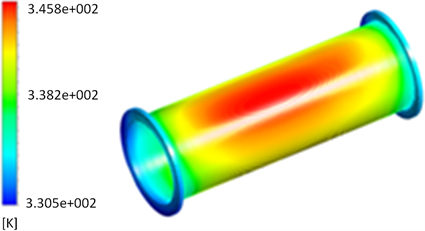(a) 10时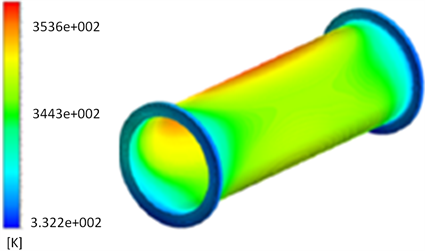(b) 14时

Figure 5. Distribution of shell temperature field distribution at different time

5.2. 风速对温度场的影响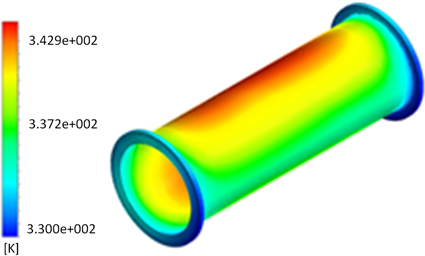(a) 2m/s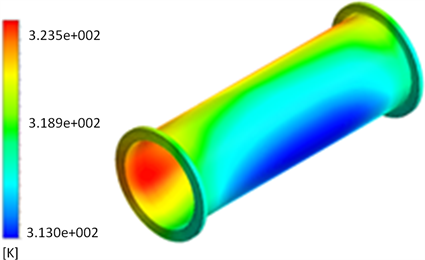(b) 10m/s

Figure 6. Distribution of shell temperature field distribution with different wind speed

6. 结论

  张施令. 220kV SF_6气体绝缘GIS真型试验平台设计与研制[J]. 水电能源科学, 2018, 36(5): 171-174+196.  吴晓文. 气体绝缘母线温升计算及监测方法的研究[D]: [博士学位论文]. 武汉: 武汉大学, 2013.  邹明翰, 缪芸. 三相共箱式气体绝缘母线热计算方法分析[J]. 水电能源科学, 2014, 32(7): 169-172+177.  谢海峰, 潘纪良, 陈湘波, 吴晓文, 王鹏. 气体绝缘母线三维涡流场有限元分析研究[J]. 陕西电力, 2016, 44(8): 12-16.  孙国霞, 舒乃秋, 吴晓文, 谢志杨, 金向朝, 彭辉. 基于多物理场耦合的气体绝缘母线触头接触温升有限元计算[J]. 电工技术学报, 2013, 28(S2): 408-413.  李洪涛. 气体绝缘母线接头过热性故障机理的研究[D]: [博士学位论文]. 武汉: 武汉大学, 2014.  Hernandez, L., Baladrón, C., Aguiar, J., et al. (2013) Short-Term Load Forecasting for Microgrids Based on Artificial Neural Networks. Energies, 6, 1385-1408. https://doi.org/10.3390/en6031385  贺兴容, 刘强, 刘守豹, 彭东. 基于三维涡流场计算的换流站PLC避雷器发热分析[J]. 电瓷避雷器, 2017(6): 156-160.  Vachirasricirikul, S. and Ngamroo, I. (2011) Robust Controller Design of Heat Pump and Plug-In Hybrid Electric Vehicle for Frequency Control in a Smart Microgrid Based on Specified-Structure Mixed H2/H∞ Control Technique. Applied Energy, 88, 3860-3868. https://doi.org/10.1016/j.apenergy.2011.04.055  舒圣浪. 基于有限公式法的永磁电机温度场与流体场计算研究[D]: [硕士学位论文]. 沈阳: 沈阳工业大学, 2016.  Berg, S. and Van Wunnik, J. (2017) Shear Rate Determination from Pore-Scale Flow Fields. Transport in Porous Media, 117, 229-246. https://doi.org/10.1007/s11242-017-0830-3  Sidorov, O.Y. and Aristova, N.A. (2017) Gas Combustion in the Heating Channel of a Coke Oven. Coke and Chemistry, 60, 316-321. https://doi.org/10.3103/S1068364X17080063  黄张伟. 基于流固耦合理论的涡轮增压器涡轮箱有限元分析[D]: [硕士学位论文]. 长沙: 湖南大学, 2014.  许红军, 曹晏飞, 李彦荣, 高杰, 蒋卫杰, 邹志荣. 日光温室太阳辐射模型构建及应用[J]. 农业工程学报, 2019, 35(7): 160-169.  闫国臣, 李先立, 李小荷. 风力等级、风速与风压的对应关系研究[J]. 门窗, 2014(8): 56-57.  付丽荣. 对流-辐射耦合传热模拟及其在柴油机缸内过程应用研究[D]: [博士学位论文]. 哈尔滨: 哈尔滨工程大学, 2016.